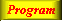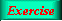Lecture 3 - Page 21 : 42
 Functional Programming in SchemeName binding, Recursion, Iteration, and Continuations * Name binding constructs The let name binding expression The equivalent meaning of let Examples with let name binding The let* name binding construct An example with let* The letrec namebinding construct LAML time functions * Conditional expressions Conditional expressions Examples with if Example with cond: leap-year? Example with cond: american-time Example with cond: as-string * Recursion and iteration Recursion List processing Tree processing (1) Tree processing (2) Recursion versus iteration Example of recursion: number-interval Examples of recursion: string-merge Examples with recursion: string-of-char-list? Exercises * Example of recursion: Hilbert Curves Hilbert Curves Building Hilbert Curves of order 1 Building Hilbert Curves of order 2 Building Hilbert Curves of order 3 Building Hilbert Curves of order 4 A program making Hilbert Curves * Continuations Introduction and motivation The catch and throw idea A catch and throw example The intuition behind continuations Being more precise The capturing of continuations Capturing, storing, and applying continuations Use of continuations for escaping purposes Practical example: Length of an improper list Practical example: Searching a binary tree
 Example of recursion: number-interval
 The function number-interval returns a list of integers from a lower bound to an upper bound
 ```(define (number-interval f t) (if (<= f t) (cons f (number-interval (+ f 1) t)) '()))```

The function number-interval from the general LAML library. This function returns a list of t-f+1 numbers from f to t .Try it out!.The function number-interval-iter is an iterative, tail recursive variant of number-interval.A sample dialogue with the number interval functions.The append functionA list replication function